python学习 . 2019/12/22发布 . shanyonggang_web . . 396阅读

• jupyter
• numpy
• pandas
• matplotlib

### 代码实现

``````import numpy as np
import pandas as pd
# 计算存活天数
def cal_live_time(start_time,end_time):
import datetime
d1 = datetime.datetime.strptime(start_time,'%Y-%m-%d')
d2 = datetime.datetime.strptime(end_time,'%Y-%m-%d')
days = (d2-d1).days
return days
need_data = df_data.loc[:,['com_name','com_born','com_change_close_date','com_style','com_position','com_tags','com_fund_status_name','com_invsts','death_reason']]
# 计算所有公司的存活时间
f = lambda x:cal_live_time(x.com_born,x.com_change_close_date)
need_data['live_time'] = need_data.apply(f,axis=1)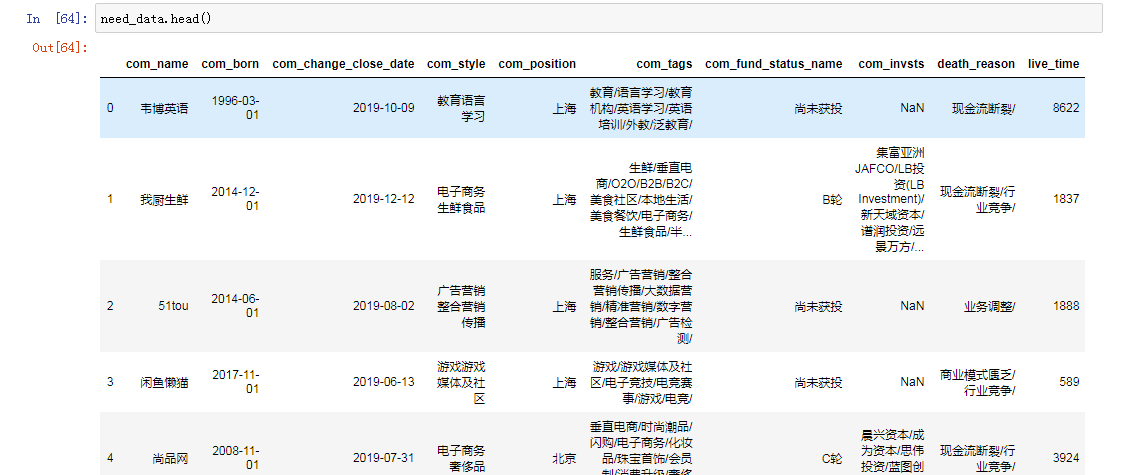``````# 获取生存时间最短的公司
need_data.loc[need_data['live_time'].idxmin(),:]
# 获取生存时间最长的公司
need_data.loc[need_data['live_time'].idxmax(),:]
# 平均存活时间
need_data['live_time'].mean()``````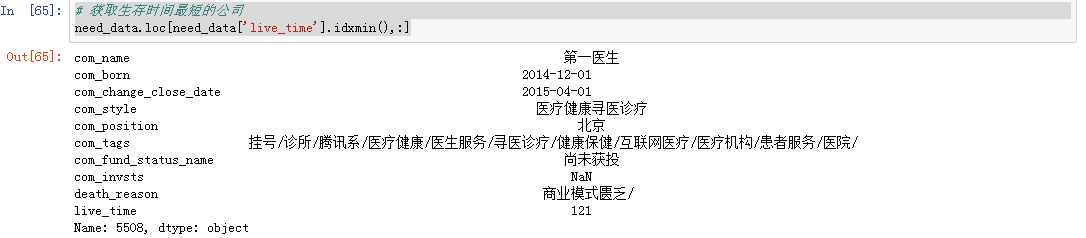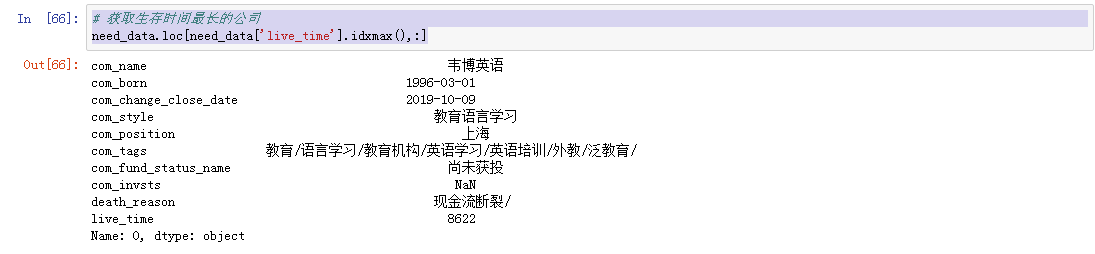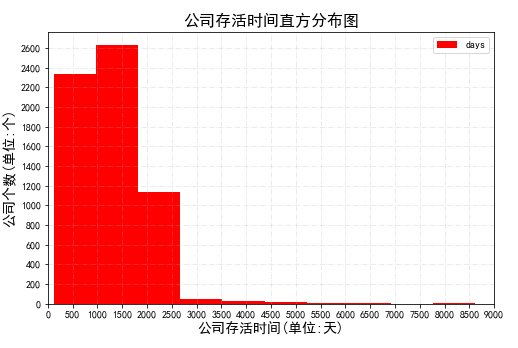``````# 统计所有公司位置的总数
com_position = need_data['com_position'].value_counts()
print(com_position)
name = np.array(list(com_position.index))
num = np.array(list(com_position.values))
plt.figure(figsize=(16,6))
plt.bar(name,num,color='rgby')
plt.title('死亡公司分布情况(TOP10)',fontsize=20)
plt.xlabel('公司地理位置',fontsize=16)
plt.ylabel('公司数量',fontsize=16)
plt.savefig('.\可视化图表\com_position.jpg')
plt.show()``````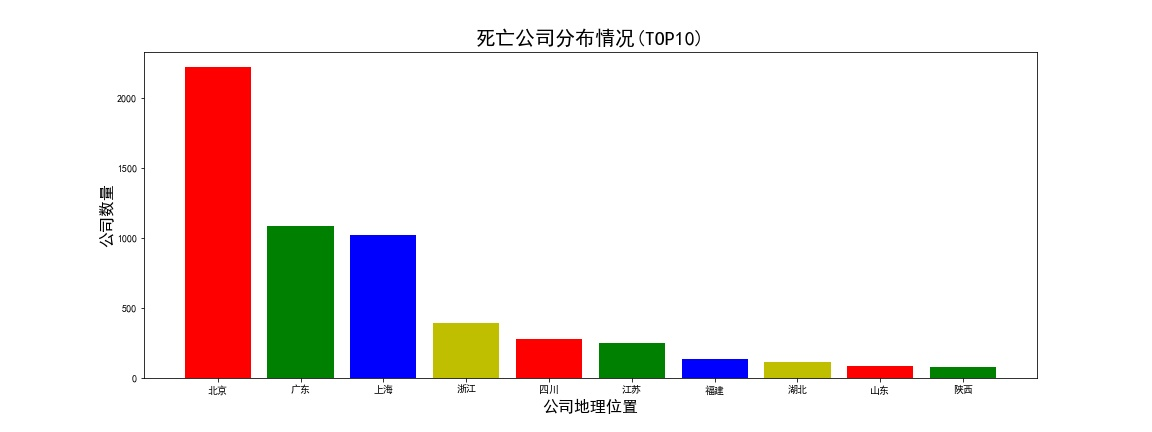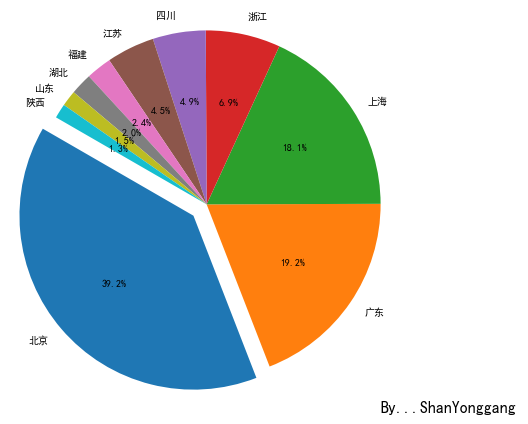``````# 公司融资情况
com_financing = need_data['com_fund_status_name'].value_counts()
print(com_financing)
plt.figure(figsize=(16,6))
# plt.bar(index,num,color='rgby')
plt.title('死亡公司融资分布情况(TOP10)',fontsize=20)
plt.xlabel('公司数量',fontsize=16)
plt.ylabel('公司融资情况',fontsize=16)
plt.savefig('.\可视化图表\com_financing.jpg')
plt.show()``````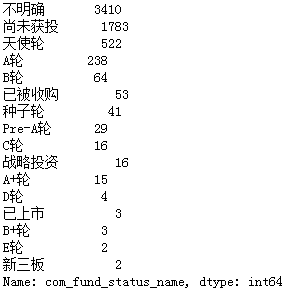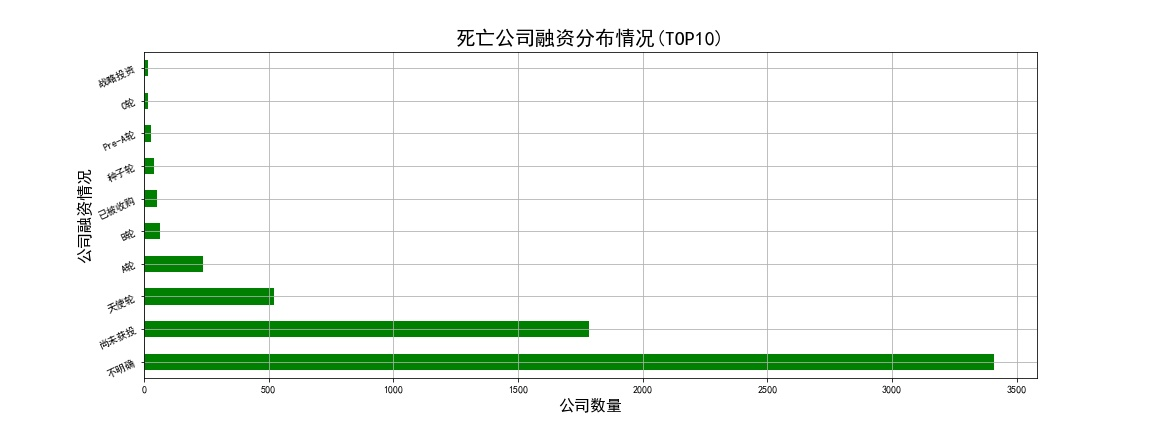``need_data[need_data['com_fund_status_name'] == '已上市']``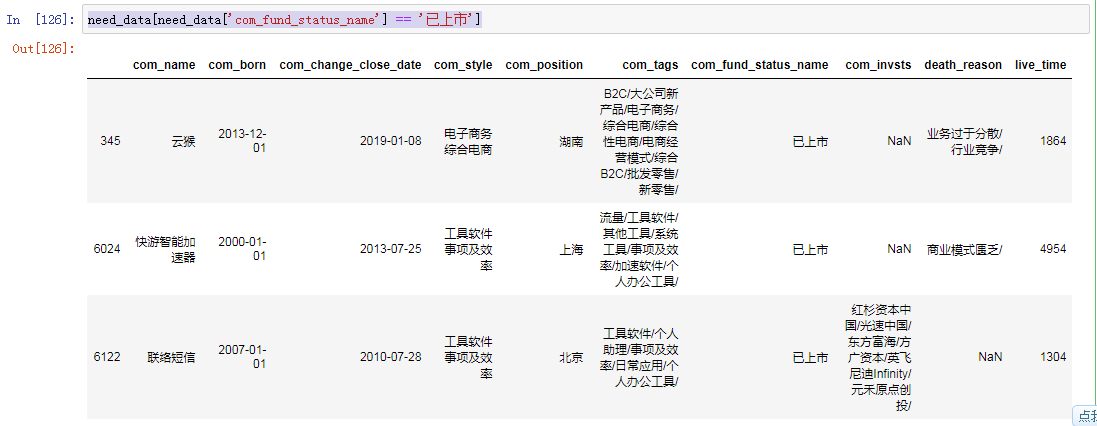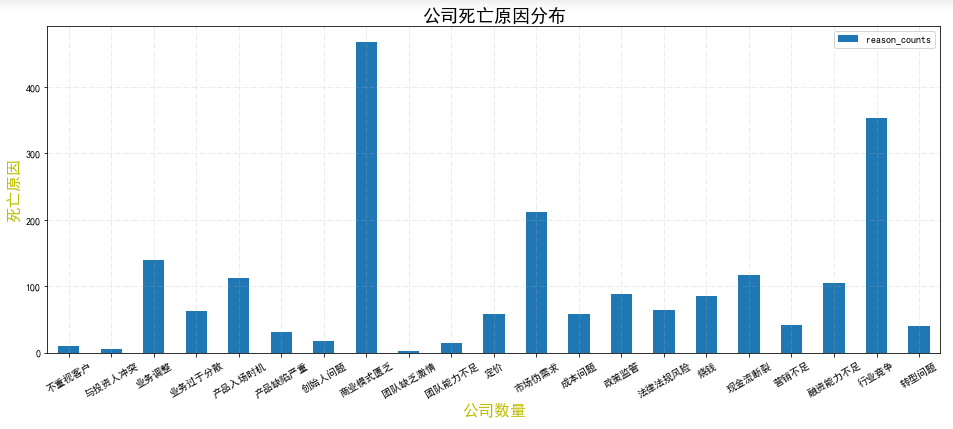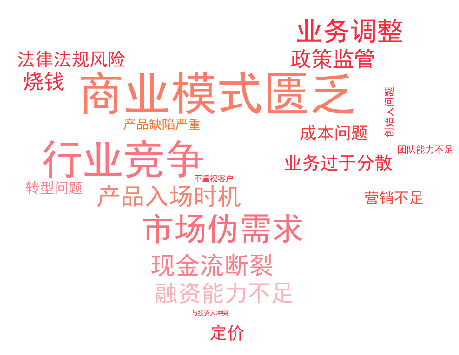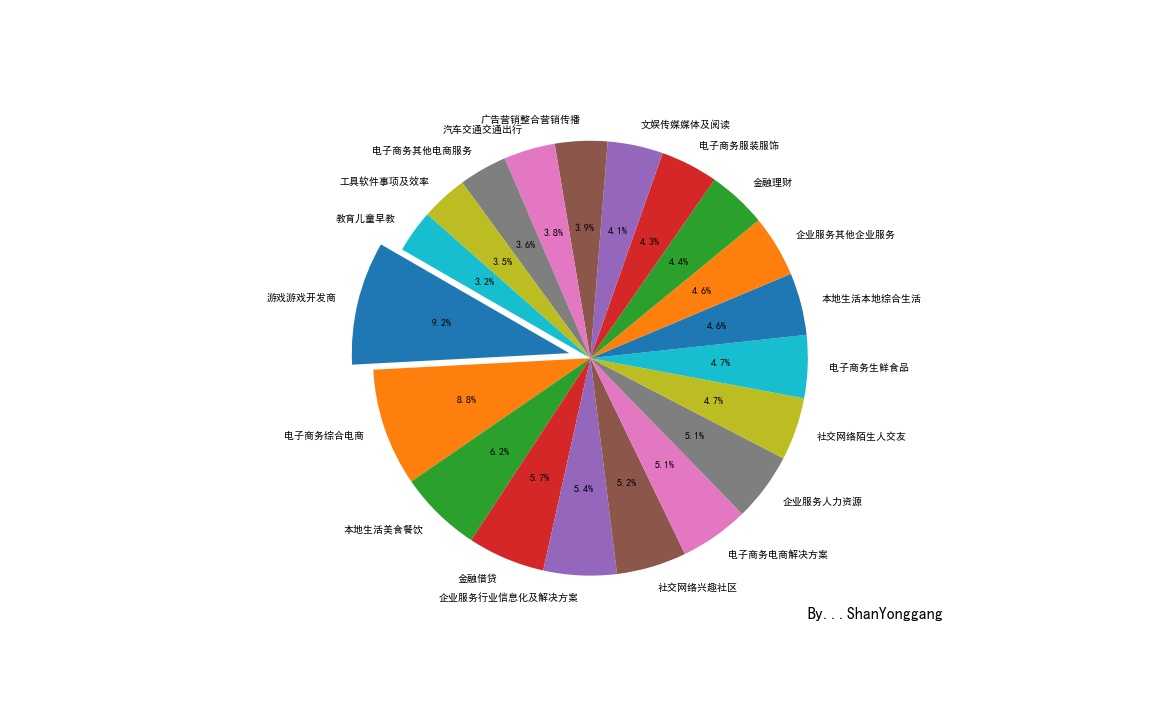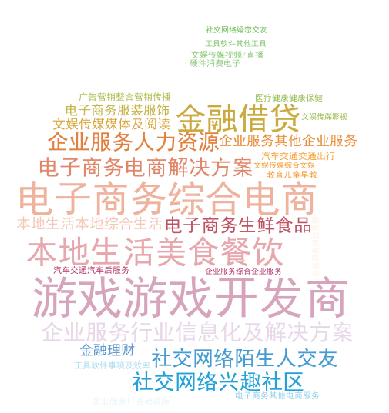``need_data[need_data['com_style']=='金融借贷'].head(20)``

``````#  统计公司标签，绘制词云图
com_tags = need_data['com_tags']
tags_total = ''
for x in com_tags:
tags_total += str(x)
tags_list = tags_total.strip('').split('/')
tags = pd.Series(tags_list).value_counts()
print(tags[:30])
fontpath = 'simhei.ttf'

wc = WordCloud(font_path = fontpath,          #设置字体
background_color = "white",    #背景颜色
max_words = 1000,              #词云显示的最大词数
max_font_size = 100,           #字体最大值
min_font_size = 10,            #字体最小值
random_state = 42,             #随机数
collocations = False,          #避免重复单词
width = 140, height = 80,    #图像宽高，需配合plt.figure(dpi=xx)放缩才有效
margin = 2                     #字间距
)
word_frequence = dict(tags)
cl = wc.generate_from_frequencies(word_frequence)
# plt.imshow(cl)
plt.figure(dpi = 140)
plt.imshow(wc.recolor(color_func=image_colors)) # 重新上色
plt.axis('off')``````### 总结

• 数据有更多可以深究和探索的地方，本文暂时只对数据进行简单的处理分析
• 后续加强numpy、pandas、matplotlib的理解与学习
• 接触算法去吧，少年！

• 有疑问请在下方评论区留言，我会尽快回复。
• Email私信我： 511248513@qq.com 或添加博主 微信